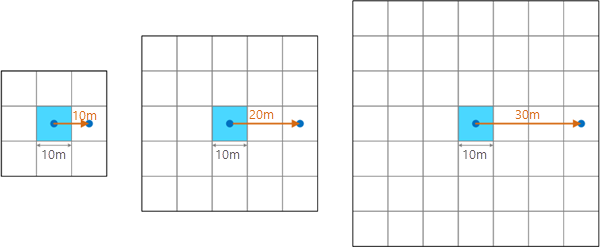# 表面参数的工作原理

## 坡向应用

• 在寻找最适合滑雪的山坡的过程中，查找某座山所有朝北的坡。
• 在统计各地区生物多样性的研究中，计算某区域中各个位置的日照强度。
• 作为判断最先遭受洪流袭击的居住区位置研究的一部分，在某山区中查找所有朝南的山坡，从而判断出雪最先融化的位置。

## 剖面（法向坡度线）曲率

• 其中：

KP = 剖面（法向坡度线）曲率

z = f(x,y)

## 切向（标准等高线）曲率

• 其中：

KT = 切向（法向等值线）曲率

z = f(x,y)

## 平面（投影等值线）曲率

• 其中：

KPC = 平面（投影等值线）曲率

z = f(x,y)

• 其中：

τ = 等值测地线扭转

z = f(x,y)

• 其中：

KM = 平均曲率

z = f(x,y)

• 其中：

KG = 高斯曲率

z = f(x,y)

## Casorati 曲率

Casorati 曲率表面参数用于测量表面的总曲率。 该值可为零或任意其他正数。 高正值表示在多个方向上的尖锐弯曲区域。

• 其中：

KC = Casorati 曲率

z = f(x,y)

## 基本曲率和组合曲率类型

• 其中：

KM = 平均曲率

KT = 切向（法向等值线）曲率

KP = 剖面（法向坡度线）曲率

z = f(x,y)

• 其中：

KG = 高斯曲率

KT = 切向（法向等值线）曲率

KP = 剖面（法向坡度线）曲率

τ = 等值测地线扭转

z = f(x,y)

• 其中：

KC = Casorati 曲率

KM = 平均曲率

KG = 高斯曲率

z = f(x,y)

## 邻域距离将显示邻域距离（橙色线）和移动窗口的像素数之间的关系。 像元大小为 10 米时；10 米的邻域距离将使用 3 x 3 像元窗口（此为默认设置），20 米的邻域距离将使用 5 x 5 像元窗口，而 30 米的邻域距离将使用 7 x 7 像元窗口。

## 测地线坐标转换

ECEF 坐标系是以地心为原点的 3D 右手笛卡尔坐标系，其中所有位置均由 X、Y 和 Z 坐标表示。 有关以地心坐标系表示的目标位置 T 的示例，请参见下图：

``X = (N(φ) + h) * cos(φ) * cos(λ)``

``Y = (N(φ) + h) * cos(φ) * sin(λ)``

``Z = (b2 / a2 * N(φ) + h) * sin(φ)``

• 其中：

``N(φ) = a2 / √( a2 * cos(φ)2 + b2 * sin(φ)2)``

φ = 纬度

λ = 经度

h = 椭球体高度

a = 椭球体的长轴

b = 椭球体的短轴

## 参考资料

B. Hofmann-Wellenhof, H. Lichtenegger and J. Collins, 2001. GPS - theory and practice. Section 10.2.1. p. 282.

Burrough, P. A., and McDonell, R. A., 1998. Principles of Geographical Information Systems (Oxford University Press, New York), 190 pp.

Crane K., 2018. Discrete Differential Geometry: An Applied Introduction. Notices of the AMS, Communication. https://www.cs.cmu.edu/~kmcrane/Projects/DDG/paper.pdf

David Eberly 1999. Least Squares Fitting of Data (Geometric Tools, LLC), pp. 3.

E.J.Krakiwsky, and D.E.Wells, 1971. Coordinate Systems In Geodesy (GEODESY AND GEOMATICS ENGINEERING, UNB), LECTURE NOTES, No16, 1971, pp. 18-38

Hengl T. and Reuter H. 2008. Geomorphometry Concepts, Software, Applications. Elsevier.

James D.E., M.D. Tomer, S.A. Porter. 2014. Trans-scalar landform segmentation from high-resolution digital elevation models. 海报发表于：ESRI 年度用户大会；2014 年 7 月；加利福尼亚州圣地亚哥。

Lancaster, P. and Šalkauskas, K. Curve and Surface Fitting: An Introduction. London: Academic Press, 1986.

Marcin Ligas, and Piotr Banasik, 2011. Conversion between Cartesian and geodetic coordinates on a rotational ellipsoid by solving a system of nonlinear equations (GEODESY AND CARTOGRAPHY), Vol. 60, No 2, 2011, pp. 145-159

Minár, J., Evans, I. S., & Jenčo, M. (2020). A comprehensive system of definitions of land surface (topographic) curvatures, with implications for their application in geoscience modelling and prediction. Earth-Science Reviews, 103414. https://doi.org/10.1016/j.earscirev.2020.103414

Wilson J.P and Gallant, J.C. (Eds.) 2000. Terrain Analysis: Principles and Applications. John Wiley & Sons, Inc.

Wilson J.P 2018. Environmental Application of Digital Terrain Modeling. John-Blackwell, Inc.

Zevenbergen, L. W., and C. R. Thorne. 1987. Quantitative Analysis of Land Surface Topography. Earth Surface Processes and Landforms 12: 47–56.